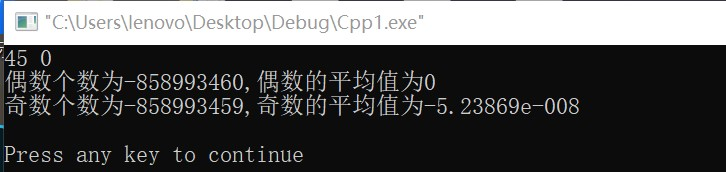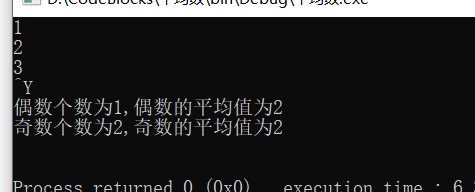2021-08-17 15:54

编写一个程序，读取整数，直到输入0结束。输入后，程序应报告用户输入的偶数（不包括0）个数，平均数，奇数的个数和输入的平均数。为什么我输入后，图片显示的内容不对，哪里出了错误啊？

#include<stdio.h>
int main()
{
int i=0;
int j;
int o;
int sum1=0,sum2=0;
while(scanf("%d",&i))
{
if(i==0)
break;
else    if(i%2==0)
{
sum1=sum1+i;
o++;
}
else
{
sum2=sum2+i;
j++;
}
}
printf("偶数个数为%d,偶数的平均值为%g\n",o,1.0*sum1/o);
printf("奇数个数为%d,奇数的平均值为%g\n",j,1.0*sum2/j);
printf("\n");
return 0;
}• 写回答
• 好问题 提建议
• 追加酬金
• 关注问题
• 收藏
• 邀请回答

1条回答默认 最新

•已采纳

o，j这个变量没有初始化为0，所以最后输出也不对
给你改好了：

#include<stdio.h>
int main()
{
int i=0;
int j=0;
int o=0;
int sum1=0,sum2=0;
while(scanf("%d",&i))
{
if(i==0)
break;
else    if(i%2==0)
{
sum1=sum1+i;
o++;
}
else
{
sum2=sum2+i;
j++;
}
}
printf("偶数个数为%d,偶数的平均值为%g\n",o,1.0*sum1/o);
printf("奇数个数为%d,奇数的平均值为%g\n",j,1.0*sum2/j);
printf("\n");
return 0;
}评论
解决 1 无用
打赏 举报